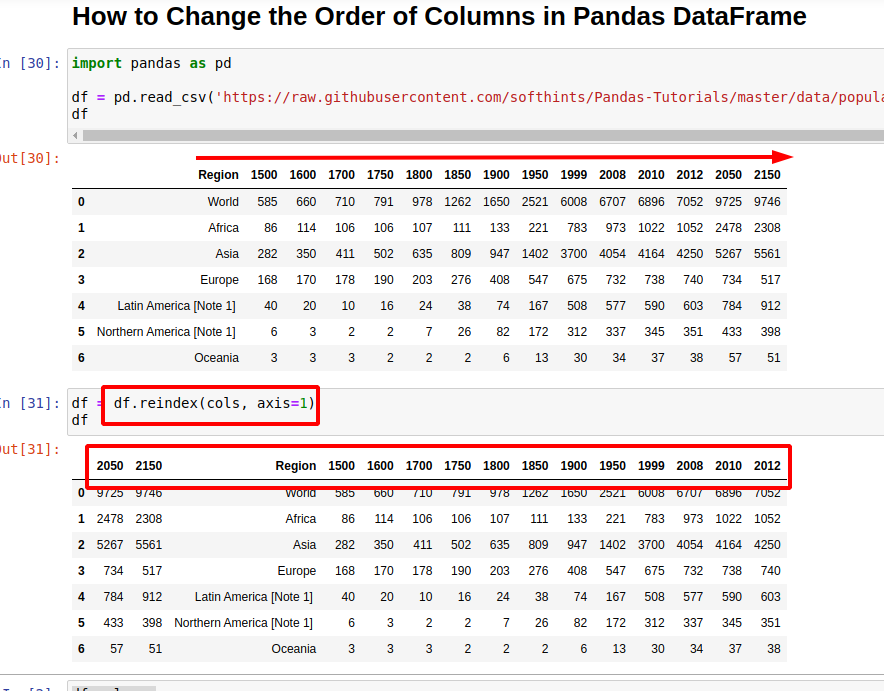Here are two ways to sort or change the order of columns in Pandas DataFrame.

(1) Use method `reindex` - custom sorts

``````df = df.reindex(sorted(df.columns), axis=1)
``````

(2) Use method `sort_index` - sort with duplicate column names

``````df = df.sort_index(axis=1)
``````

What is the difference between if need to change order of columns in DataFrame : `reindex` and `sort_index`.

The `sort_index` is a bit faster (depends on data and column number) and can be used with duplicate names. `reindex` is suitable if you need to apply custom order or sorting.

Both of them work for the two axis - rows and columns,

Suppose we have data like:

Region 1500 1600 1700 1750 1800 1850 1900
World 585 660 710 791 978 1262 1650
Africa 86 114 106 106 107 111 133
Asia 282 350 411 502 635 809 947
Europe 168 170 178 190 203 276 408
Latin America [Note 1] ​ 40 20 10 16 24 38 74

Where the full list of columns is:

``````Index(['Region', '1500', '1600', '1700', '1750', '1800', '1850', '1900',
'1950', '1999', '2008', '2010', '2012', '2050', '2150'],
dtype='object')
``````

Data is available by:

``````import pandas as pd

``````

Before the change of the order let's shuffle the columns and get the initial order:

``````import random

initial_order = df.columns.to_list()
cols = df.columns.to_list()

random.shuffle(cols)
``````

## 1: Change order of columns by `reindex`

First example will show us how to use method `reindex` in order to sort the columns in alphabetical order:

``````df = df.reindex(sorted(df.columns), axis=1)
``````

By `df.columns` we get all column names as they are stored in the DataFrame. We sort them by `sorted` and finally use the method `reindex` on columns.

A shorter code to sort the columns by name would be:

``````df = df[sorted(df.columns)]
``````

### Custom sort of columns with `reindex`

In order to change the column order in a custom way we can use method `reindex`. As we saw earlier we can get the list of columns and shuffle them:

``````cols = df.columns.to_list()

random.shuffle(cols)
``````

Now we can apply this order to the DataFrame by:

``````df = df.reindex(df.columns, axis=1)
``````

The columns of the updated DataFrame:

``````Index(['1500', '1900', '2000', '1900', '1850', '2000', '2000', '1900', '1700',
'1800', '1600', '2000', '2150', '1750', 'Region'],
dtype='object')
``````## 2: Sort columns by name by method `sort_index`

Alternative solution is to use the method `sort_index`. It doesn't support custom order but it's faster in general.

To update the column order by `sort_index` use this syntax:

``````df = df.sort_index(axis=1)
``````

The official documentation for this method says:

Returns a new DataFrame sorted by label if inplace argument is False, otherwise updates the original DataFrame and returns None.

This method has parameter `inplace` - which is not the case for `reindex`.

## 3: Sort with duplicate column names

Finally let's see what will happen if we apply method `reindex` on DataFrame with duplicate column names. To achieve this we are going to update column names manually:

``````df.columns = ['1500', '1600', '1700', '1750', '1800', '1850', '1900', '1900', '1900',
'2000', '2000', '2000', '2000', '2150', 'Region']
``````

Method `reindex` is raising error:

ValueError: cannot reindex from a duplicate axis

While `sort_index` is working successfully

## 4: Shift columns in Pandas DataFrame

Finally let's see how to shift columns in Pandas DataFrame. This is possible by getting a list of columns names and updating the list of columns:

``````cols = df.columns.to_list()
cols = cols[-2:] + cols[:-2]
``````

result:

``````['2050', '2150', 'Region', '1500', '1600', '1700', '1750', '1800', '1850', '1900', '1950', '1999', '2008', '2010', '2012']
``````

Finally we can update the DataFrame order by:

``````df = df.reindex(cols, axis=1)
``````

or by:

``````df = df[cols]
``````

The `df.reindex` is the faster than the second solution

## 5: Performance comparison for `reindex` and `sort_index`

Finally lets check the performance for a pretty small DataFrame - (7, 15) between:

• `reindex` - 254 µs ± 1.84 µs per loop (mean ± std. dev. of 7 runs, 1000 loops each)
• `sort_index` - 181 µs ± 7.34 µs per loop (mean ± std. dev. of 7 runs, 1000 loops each)

The same comparison for (700000, 15):

• `reindex` - 24.1 ms ± 740 µs per loop (mean ± std. dev. of 7 runs, 10 loops each)
• `sort_index` - 22.7 ms ± 430 µs per loop (mean ± std. dev. of 7 runs, 10 loops each)

For (7, 1500):

• `reindex` - 383 µs ± 4.93 µs per loop (mean ± std. dev. of 7 runs, 1000 loops each)
• `sort_index` - 826 µs ± 11.4 µs per loop (mean ± std. dev. of 7 runs, 1000 loops each)